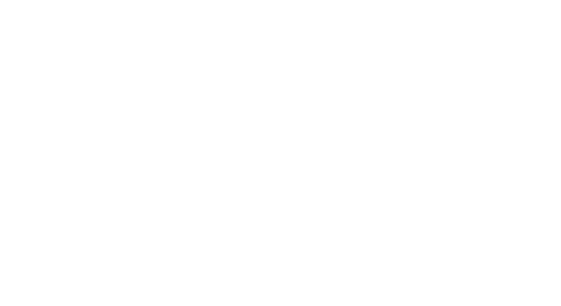Arthur Fattakhov, Arthur Kuzin, 以及 Ilya Kibrdin 是本次比赛亚军团队，他们的最终得分为 0.987。Vladimir Iglovikov 是获得第 9 名的团队的成员，最终得分为 0.985。这两支团队的解决方案非常相似，因此他们决定写一份技术报告。Ruslan 是深圳大学大数据研究院的一名研究生，他帮助完成了文本方面的工作，这对于这篇论文的顺利发表至关重要。

```1. Sony NEX-7
2. Motorola Moto X
3. Motorola Nexus 6
4. Motorola DROID MAXX
5. LG Nexus 5x
6. Apple iPhone 6
7. Apple iPhone 4s
8. HTC One M7
9. Samsung Galaxy S4
10. Samsung Galaxy Note 3```

```1. JPEG 压缩质量因子 = 70
2. JPEG 压缩质量因子 = 90
3. 通过因子 = 0.5 的双三次插值进行缩放
4. 通过因子 = 0.8 的双三次插值进行缩放
5. 通过因子 = 1.5 的双三次插值进行缩放
6. 通过因子 = 2.0 的双三次插值进行缩放
7. 使用 gamma = 0.8 的伽马校正
8. 使用 gamma = 1.2 的伽马校正```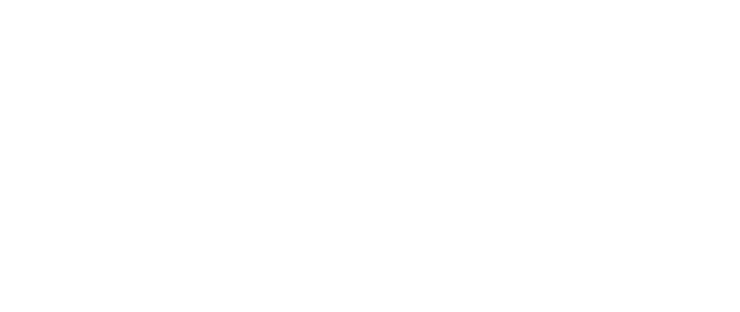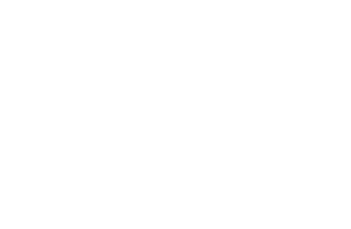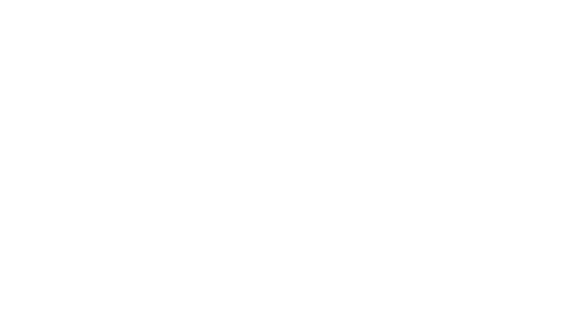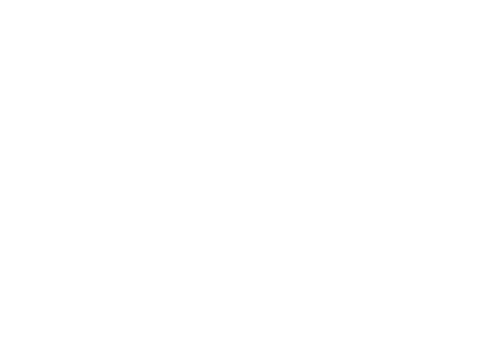## 更多的跳跃连接!

DenseNet 论文的作者将卷积块中的所有层连接起来，跳跃连接简化了梯度流，使得训练深层网络成为可能。在跳跃连接成为主流之前，处理只有 19 层的 VGG19 网络是一件痛苦的事情，但是在引入它们之后，使用具有 100 多个层的网络处理高度抽象特性就不再是一个困难的问题了。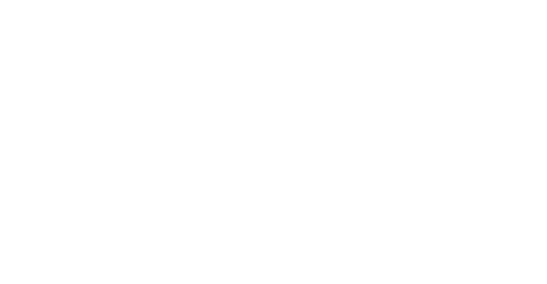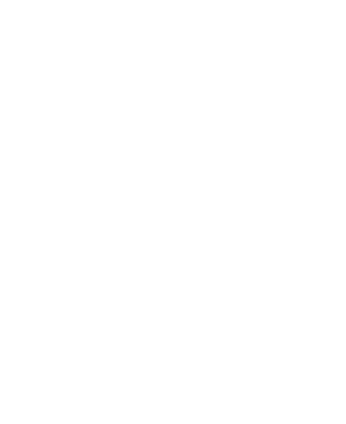DenseNet比Resnet更好/更差吗？这个问题得视情况而定。在「Planet: Understanding the Amazon from Space challenge」竞赛（https://www.kaggle.com/c/planet-understanding-the-amazon-from-space）中，我们团队使用  DenseNet，从 900 多支团队中夺得第 7 名，这一网络在这次比赛中表现得更好，但它在 ImageNet 上的表现较差。

1. Dihedral Group D4 变换：将图像旋转 90、180、270 度，以及进行翻转操作。

2. 伽马变换：我们在 [80，120] 的范围内均匀地选择了伽马参数。

3. 质量因子参数从 70 到 90 均匀采样的 JPEG 压缩。

4. 缩放因子在 [0.5，2] 的范围内的变换。

import albumentations as albu
def train_transform(image, p=1):
aug = albu.Compose([albu.RandomRotate90(p=0.5),
albu.HorizontalFlip(p=0.5),
albu.RandomGamma(gamma_limit=(80, 120), p=0.5),
albu.JpegCompression(quality_lower=70, quality_upper=90, p=0.5),
albu.RandomScale(scale_limit=(0.5, 2), interpolation=cv2.INTER_CUBIC, p=1)
], p=p)
return aug(image=image)[‘image’]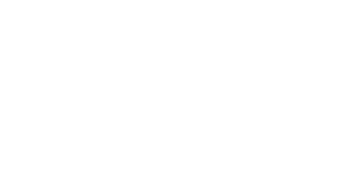import albumentations as albu
def train_transform(image, p=1):
scale_limit = (0.5, 2)    aug = albu.Compose([
albu.RandomRotate90(p=0.5),
albu.HorizontalFlip(p=0.5),
albu.RandomGamma(gamma_limit=(80, 120), p=0.5),
albu.JpegCompression(quality_lower=70, quality_upper=90, p=0.5),
albu.OneOf([albu.RandomScale(scale_limit=scale_limit, interpolation=cv2.INTER_NEAREST, p=0.5),
albu.RandomScale(scale_limit=scale_limit, interpolation=cv2.INTER_LINEAR, p=0.5),
albu.RandomScale(scale_limit=scale_limit, interpolation=cv2.INTER_CUBIC, p=0.5),
albu.RandomScale(scale_limit=scale_limit, interpolation=cv2.INTER_AREA, p=0.5),
albu.RandomScale(scale_limit=scale_limit, interpolation=cv2.INTER_LANCZOS4, p=0.5),
], p=0.5),
], p=p)
return aug(image=image)[‘image’]

import albumentations as albu
def train_transform(image, p=1):
aug = albu.Compose([
albu.RandomCrop(height=960, width=960, p=1),
albu.RandomRotate90(p=0.5),
albu.HorizontalFlip(p=0.5),
albu.RandomGamma(gamma_limit=(80, 120), p=0.5),
albu.JpegCompression(quality_lower=70, quality_upper=90, p=0.5),
albu.RandomScale(scale_limit=(0.5, 2), interpolation=cv2.INTER_CUBIC, p=1)
albu.RandomCrop(height=480, width=480, p=1),
], p=p)
return aug(image=image)[‘image’]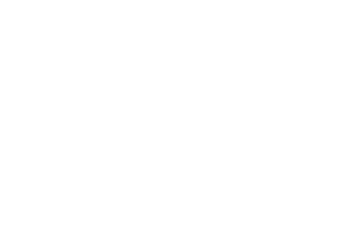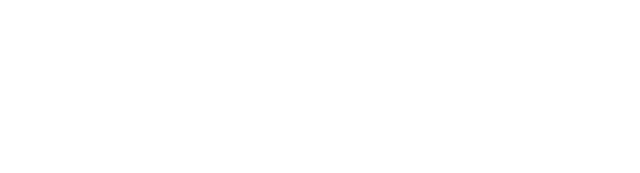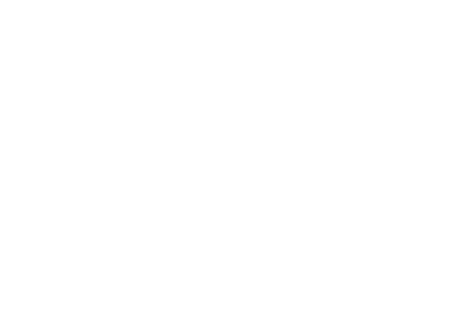## 总结：

1. 利用 ImageNet 预训练模型进行的迁移学习在相机检测等使用低级特征的任务中，可以取得很好的性能。

3. 在训练中使用数据增强技术会帮助模型提升鲁棒性。

4. 如果你能获取到更多的带标签的数据，尽量去获取吧。在大多数情况下，尽管利用领域知识可以提供额外的重要帮助，「暴力」地处理大量数据可能比使用复杂的解决方案提高模型性能更容易。

## 文中略过的内容:

1. 是否有必要添加额外的输出来显示出图像是否被修改过？

2. checkpoint averaging 等技巧。

3. 其他架构的性能如何？

4. 如何将集成学习技术应用到这个问题上。

5. 测试集中的类是平衡的，我们可以如何利用。

6. 如何将伪标记技术（一种半监督学习技术）应用于此任务。

7. 对那些我们尝试过、但是没有成功的方法进行讨论。

8. 解释网络学习到的特征是什幺？

9. 评价指标的细微差别。